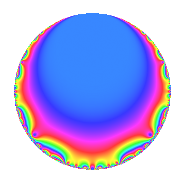Properties

 Label 83.1.bLevel 83 Weight 1 Character orbit b Rep. character $$\chi_{83}(82,\cdot)$$ Character field $$\Q$$ Dimension 1 Newforms 1 Sturm bound 7 Trace bound 0

Related objects

Defining parameters

 Level: $$N$$ = $$83$$ Weight: $$k$$ = $$1$$ Character orbit: $$[\chi]$$ = 83.b (of order $$2$$ and degree $$1$$) Character conductor: $$\operatorname{cond}(\chi)$$ = $$83$$ Character field: $$\Q$$ Newforms: $$1$$ Sturm bound: $$7$$ Trace bound: $$0$$

Dimensions

The following table gives the dimensions of various subspaces of $$M_{1}(83, [\chi])$$.

Total New Old
Modular forms 2 2 0
Cusp forms 1 1 0
Eisenstein series 1 1 0

The following table gives the dimensions of subspaces with specified projective image type.

$$D_n$$ $$A_4$$ $$S_4$$ $$A_5$$
Dimension 1 0 0 0

Trace form

 $$q$$ $$\mathstrut -\mathstrut q^{3}$$ $$\mathstrut +\mathstrut q^{4}$$ $$\mathstrut -\mathstrut q^{7}$$ $$\mathstrut +\mathstrut O(q^{10})$$ $$q$$ $$\mathstrut -\mathstrut q^{3}$$ $$\mathstrut +\mathstrut q^{4}$$ $$\mathstrut -\mathstrut q^{7}$$ $$\mathstrut -\mathstrut q^{11}$$ $$\mathstrut -\mathstrut q^{12}$$ $$\mathstrut +\mathstrut q^{16}$$ $$\mathstrut -\mathstrut q^{17}$$ $$\mathstrut +\mathstrut q^{21}$$ $$\mathstrut +\mathstrut 2q^{23}$$ $$\mathstrut +\mathstrut q^{25}$$ $$\mathstrut +\mathstrut q^{27}$$ $$\mathstrut -\mathstrut q^{28}$$ $$\mathstrut -\mathstrut q^{29}$$ $$\mathstrut -\mathstrut q^{31}$$ $$\mathstrut +\mathstrut q^{33}$$ $$\mathstrut -\mathstrut q^{37}$$ $$\mathstrut +\mathstrut 2q^{41}$$ $$\mathstrut -\mathstrut q^{44}$$ $$\mathstrut -\mathstrut q^{48}$$ $$\mathstrut +\mathstrut q^{51}$$ $$\mathstrut -\mathstrut q^{59}$$ $$\mathstrut -\mathstrut q^{61}$$ $$\mathstrut +\mathstrut q^{64}$$ $$\mathstrut -\mathstrut q^{68}$$ $$\mathstrut -\mathstrut 2q^{69}$$ $$\mathstrut -\mathstrut q^{75}$$ $$\mathstrut +\mathstrut q^{77}$$ $$\mathstrut -\mathstrut q^{81}$$ $$\mathstrut +\mathstrut q^{83}$$ $$\mathstrut +\mathstrut q^{84}$$ $$\mathstrut +\mathstrut q^{87}$$ $$\mathstrut +\mathstrut 2q^{92}$$ $$\mathstrut +\mathstrut q^{93}$$ $$\mathstrut +\mathstrut O(q^{100})$$

Decomposition of $$S_{1}^{\mathrm{new}}(83, [\chi])$$ into irreducible Hecke orbits

Label Dim. $$A$$ Field Image CM RM Traces $q$-expansion
$$a_2$$ $$a_3$$ $$a_5$$ $$a_7$$
83.1.b.a $$1$$ $$0.041$$ $$\Q$$ $$D_{3}$$ $$\Q(\sqrt{-83})$$ None $$0$$ $$-1$$ $$0$$ $$-1$$ $$q-q^{3}+q^{4}-q^{7}-q^{11}-q^{12}+q^{16}+\cdots$$